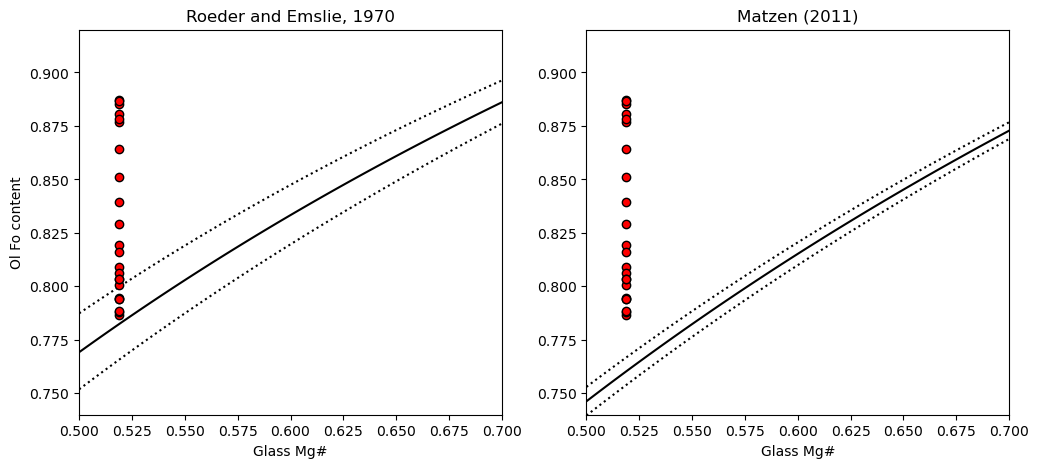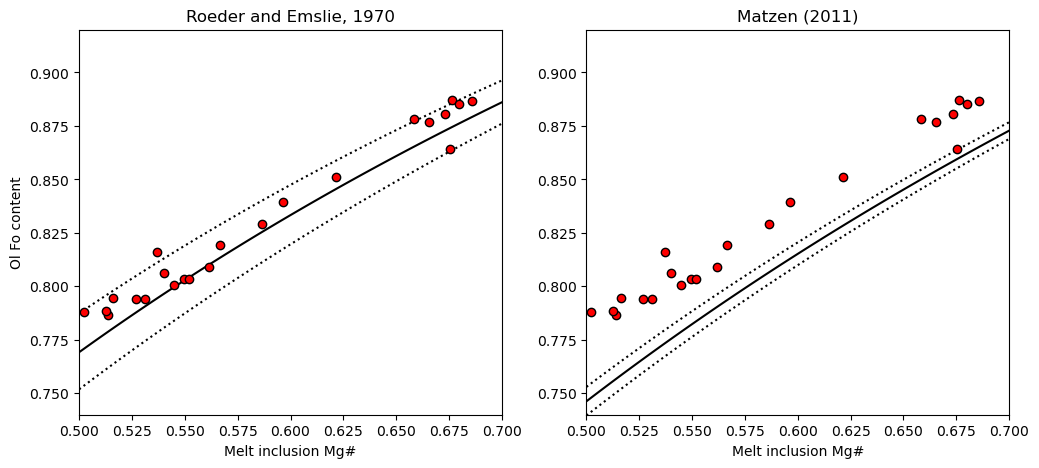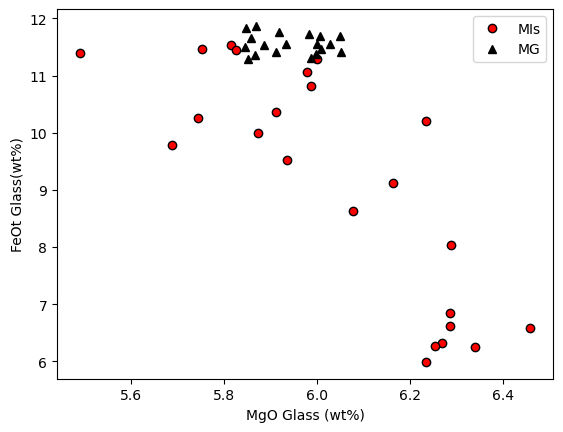This page was generated from docs/Examples/Melt_Inclusion_FeMg_Equilibrium/Ol_Melt_Inclusion_Mg_Fe_Eq_SingleSamples.ipynb. Interactive online version:.

# 1 Sample - Fe-Mg equilibrium in ol-hosted MIs

:

## Install Thermobar if you haven't already (remove the # and press run!)

#!pip install Thermobar

:

# Loading various python things
import numpy as np
import pandas as pd
import matplotlib.pyplot as plt
import Thermobar as pt


## Load in the matrix glasses

:

# We dont have to add _Liq as its all liquids,
# just specify the _Liq in the import
MG_input=pt.import_excel('2018_MIs_Glasses.xlsx',
sheet_name='LL4_MGs', suffix="_Liq")
MG_all=MG_input['my_input'] ## All columns
MG_Liqs=MG_input['Liqs'] ## Just Liquid columns

:

SiO2_Liq TiO2_Liq Al2O3_Liq FeOt_Liq MnO_Liq MgO_Liq CaO_Liq Na2O_Liq K2O_Liq Cr2O3_Liq P2O5_Liq H2O_Liq Fe3Fet_Liq NiO_Liq CoO_Liq CO2_Liq Sample_ID_Liq
0 50.6243 2.8773 13.0360 11.8288 0.1830 5.8471 10.2471 2.5120 0.5234 0.0 0.2689 0.0 0.0 0.0 0.0 0.0 0
1 50.0975 2.8840 12.8885 11.2897 0.1769 5.8521 9.9658 2.4681 0.5305 0.0 0.2652 0.0 0.0 0.0 0.0 0.0 1
2 50.1346 2.8741 12.8953 11.8696 0.1814 5.8682 10.2012 2.4618 0.5778 0.0 0.2875 0.0 0.0 0.0 0.0 0.0 2
3 50.5233 2.8765 12.9818 11.3536 0.1454 5.8673 10.1806 2.6206 0.5206 0.0 0.2759 0.0 0.0 0.0 0.0 0.0 3
4 51.5840 2.9055 13.3290 11.5014 0.2286 5.8450 10.0408 2.1722 0.5565 0.0 0.2693 0.0 0.0 0.0 0.0 0.0 4

## Load in the melt inclusion data and the olivines

• As they are in the same sheet, we have to make sure we have _Liq after the melt inclusion oxide, and _Ol after the olivine

:

MIs_in=pt.import_excel('2018_MIs_Glasses.xlsx',
sheet_name='LL4_MIs')
MIs_Ol=MIs_in['Ols']
MIs_Liqs=MIs_in['Liqs']
MIs_input=MIs_in['my_input']


## Workflow 1: Assess if the olivines are in equilibrium with the co-erupted matrix glass

### 1. Calculate Mg# for liquids

:

Liq_Mgno_calc=pt.calculate_liq_mgno(liq_comps=MG_Liqs, Fe3Fet_Liq=0.15)
# Calculate mean value to plot
Liq_Mgno_calc_mean=np.mean(Liq_Mgno_calc)


### 2. Calculate Olivine Fo contents

:

Ol_Fo_Calc=pt.calculate_ol_fo(ol_comps=MIs_Ol)


### 3. Calculate fields you want to plot on a Rhodes diagram

:

Rhodes=pt.calculate_ol_rhodes_diagram_lines(Min_Mgno=0.5, Max_Mgno=0.7)

:

Mg#_Liq Eq_Ol_Fo_Roeder (Kd=0.3) Eq_Ol_Fo_Roeder (Kd=0.27) Eq_Ol_Fo_Roeder (Kd=0.33) Eq_Ol_Fo_Matzen (Kd=0.34) Eq_Ol_Fo_Matzen (Kd=0.328) Eq_Ol_Fo_Matzen (Kd=0.352)
0 0.500000 0.769231 0.787402 0.751880 0.746269 0.753012 0.739645
1 0.502020 0.770662 0.788751 0.753384 0.747796 0.754512 0.741198
2 0.504040 0.772087 0.790095 0.754883 0.749317 0.756006 0.742745
3 0.506061 0.773506 0.791432 0.756375 0.750832 0.757493 0.744286
4 0.508081 0.774919 0.792763 0.757861 0.752341 0.758975 0.745822

### 4. Plot the Kd model you want, along with the olivine and glass Mg

:

fig, (ax1, ax2) = plt.subplots(1, 2, figsize=(12,5))

# Plotting for Roeder and Emslie
ax1.set_title('Roeder and Emslie, 1970')
# Plotting equilibrium lines
ax1.plot(Rhodes['Mg#_Liq'], Rhodes['Eq_Ol_Fo_Roeder (Kd=0.27)'], ':k')
ax1.plot(Rhodes['Mg#_Liq'], Rhodes['Eq_Ol_Fo_Roeder (Kd=0.33)'], ':k')
ax1.plot(Rhodes['Mg#_Liq'], Rhodes['Eq_Ol_Fo_Roeder (Kd=0.3)'], '-k')

# Plotting data
ax1.plot(Ol_Fo_Calc*0+Liq_Mgno_calc_mean, Ol_Fo_Calc, 'ok', mfc='red')

ax2.set_title('Matzen (2011)')
# Plotting equilibrium lines
ax2.plot(Rhodes['Mg#_Liq'], Rhodes['Eq_Ol_Fo_Matzen (Kd=0.328)'], ':k')
ax2.plot(Rhodes['Mg#_Liq'], Rhodes['Eq_Ol_Fo_Matzen (Kd=0.352)'], ':k')
ax2.plot(Rhodes['Mg#_Liq'], Rhodes['Eq_Ol_Fo_Matzen (Kd=0.34)'], '-k')
# Plotting data
ax2.plot(Ol_Fo_Calc*0+Liq_Mgno_calc_mean, Ol_Fo_Calc, 'ok', mfc='red')
ax1.set_ylabel('Ol Fo content')
ax1.set_xlabel('Glass Mg#')
ax2.set_xlabel('Glass Mg#')
ax1.set_ylim([0.74, 0.92])
ax2.set_ylim([0.74, 0.92])
ax1.set_xlim([0.5, 0.7])
ax2.set_xlim([0.5, 0.7])

:

(0.5, 0.7)• We can see that a lot of the olivines are way to primitive to be in equilibrium with the co-erupted liquid, regardless of what Kd model we use. This is the first sign that the olivines have undergone extensive post-entrapment cooling and crystallization.

## Workflow 2: Assess if the olivines and their melt inclusions are in equilibrium

• If post entrapment crystallization occurs, then the crystals erupt immediatly, the melt inclusion and host olivine will be out of Mg-Fe equilibirum. -However, as Danysuhevsky et al. show in a series of papers if sufficient time passes between the cooling event driving PEC and eruption, Fe-Mg will re-equilbrate, Fe will be lost from the inclusion, and the melt inclusion will re-approach equilibrium with its host olivine

## 1. Calculate melt inclusion Mg

:

MI_Mgno_calc=pt.calculate_liq_mgno(liq_comps=MIs_Liqs, Fe3Fet_Liq=0.15)
# Calculate mean value to plot
MI_Mgno_calc_mean=np.mean(MI_Mgno_calc)


## 2. Plot on a rhodes diagram

:

fig, (ax1, ax2) = plt.subplots(1, 2, figsize=(12,5))

# Plotting for Roeder and Emslie
ax1.set_title('Roeder and Emslie, 1970')
# Plotting equilibrium lines
ax1.plot(Rhodes['Mg#_Liq'], Rhodes['Eq_Ol_Fo_Roeder (Kd=0.27)'], ':k')
ax1.plot(Rhodes['Mg#_Liq'], Rhodes['Eq_Ol_Fo_Roeder (Kd=0.33)'], ':k')
ax1.plot(Rhodes['Mg#_Liq'], Rhodes['Eq_Ol_Fo_Roeder (Kd=0.3)'], '-k')

# Plotting data
ax1.plot(MI_Mgno_calc, Ol_Fo_Calc, 'ok', mfc='red')

ax2.set_title('Matzen (2011)')
# Plotting equilibrium lines
ax2.plot(Rhodes['Mg#_Liq'], Rhodes['Eq_Ol_Fo_Matzen (Kd=0.328)'], ':k')
ax2.plot(Rhodes['Mg#_Liq'], Rhodes['Eq_Ol_Fo_Matzen (Kd=0.352)'], ':k')
ax2.plot(Rhodes['Mg#_Liq'], Rhodes['Eq_Ol_Fo_Matzen (Kd=0.34)'], '-k')
# Plotting data
ax2.plot(MI_Mgno_calc, Ol_Fo_Calc, 'ok', mfc='red')
ax1.set_ylabel('Ol Fo content')
ax1.set_xlabel('Melt inclusion Mg#')
ax2.set_xlabel('Melt inclusion Mg#')
ax1.set_ylim([0.74, 0.92])
ax2.set_ylim([0.74, 0.92])
ax1.set_xlim([0.5, 0.7])
ax2.set_xlim([0.5, 0.7])

:

(0.5, 0.7)• We can see using the Roeder and Emslie model, the melt inclusions are perfectly in line with the equilibrium field. Combined with the extensive olivine-matrix glass Fe-Mg disequilibrium, this shows extensive PEC, followed by diffusive re-equilibration

• Another line of evidence comes from plotting matrix glass and melt inclusoins in MgO-FeO space, its clear MI have lost abundant Fe

:

plt.plot(MIs_Liqs['MgO_Liq'], MIs_Liqs['FeOt_Liq'], 'ok', mfc='red', label='MIs')
plt.plot(MG_Liqs['MgO_Liq'], MG_Liqs['FeOt_Liq'], '^k', mfc='k', label='MG')
plt.xlabel('MgO Glass (wt%)')
plt.ylabel('FeOt Glass(wt%)')
plt.legend()

:

<matplotlib.legend.Legend at 0x1eb6b985ca0>